AA3RL Transmission Line Calculator

Introduction:

There are a variety of programs available to the Radio Amateur that will calculate virtually every transmission line parameter that one may need. However, I have found the routines to be inconveniently scattered among many different programs. In addition, the programs usually require the data to be entered in serial form in order to obtain the calculated output. If another calculation is desired, all the data must be re-entered. As a result, I felt the need to create a spreadsheet style program where all input values are displayed and multiple "what-if" data changes may be implemented quickly with the click of the mouse.

Initially I used the spreadsheet to design Stub and Series Section Line Matches. Since then other utility functions were added that has enabled it to replace the Smith Chart, the pocket calculator, and the many computer programs for transmission line design applications. I hope you will find it equally useful.

Caution:

All formulas assume lossless transmission lines, but these results are usually within the measurement tolerance achievable by the amateur in normal situations. However, as line loss increases, i.e. with high SWR or long transmission line lengths, these approximations will diverge from the actual values. An excellent program to check the results for lossy lines is TLA, which is included with recent versions of the ARRL Antenna Book.

Format:

The spreadsheet is divided into convenient functional areas. Each area performs one of the calculations listed below. All sections share a common layout. The input data is grouped on the left side of the page, and the output data is grouped to its immediate right. Output values are shown in bold type. On the far right side of each section there are areas that are used for computational purposes, in order to break complex formulas into more manageable chunks. If these cells are disturbed or over-written the calculations may be cease to function properly. I have learned from experience to keep a backup copy of the spreadsheet for when this eventually happens.

Contents:

The program performs the following calculations:
Length Conversion: Wavelength, Degrees, Feet
Series and Parallel Equivalent values
Capacitance and Inductance required for given reactance (X)
Air Wound Coil construction
Reactance and Length of Stubs
Stub Matching - Impedance (Z) over a 180 degree cycle along a lossless transmission line.
Impedance (Z) at any single point on a lossless transmission line (for stub matching)
Series Section Line Transformer (analytic solution) for impedance matching

Length Conversion: Wavelength, Degrees, Feet

In the screen shot below, we want to make a transmission line 1/8 (0.125) wavelength long, using 50 ohm line with a velocity factor of 0.78, and operating at a frequency of 7.0 MHz. This data is entered on the left side. The output indicates that the length should be 13.7 feet, which is 45 degrees. Alternatively, the reverse calculations may be performed.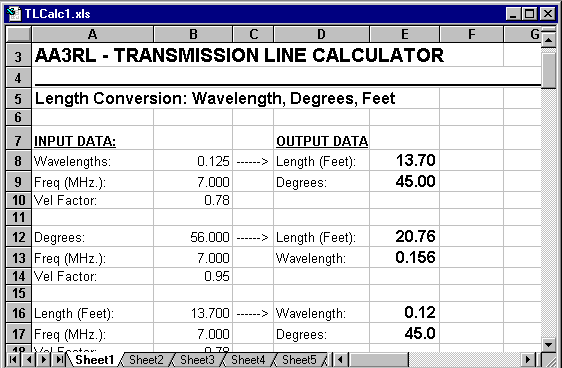Series and Parallel Equivalent values

Series and Parallel equivalent circuits are very useful for transmission line matching, as well as other circuit design applications. Conversions may be made in either direction.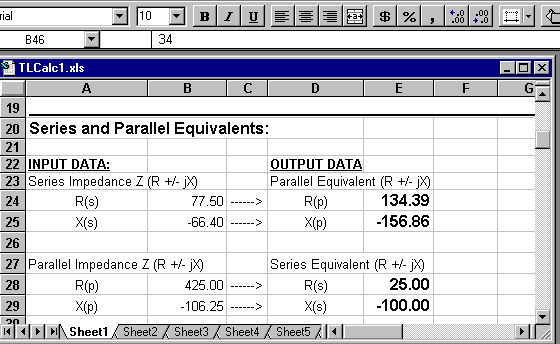Capacitance and Inductance required for given reactance (X)

At any given frequency, input the desired inductive reactance (+X) or capacitive reactance (-X). The spreadsheet calculates the required inductance (uH.) or capacitance (uF and pf).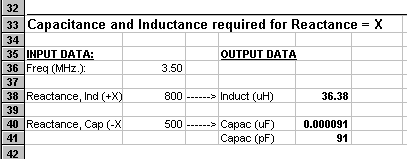Reactance and Length of Stubs

Another way to obtain a desired reactance is with the use of transmission line stubs. Input the desired inductive reactance (+X) or capacitive reactance (-X). The spreadsheet calculates the required length of stub: open for -X, shorted for +X.

Alternatively, given a stub of any length in feet which is less than ¼ wavelength, the reactance will be calculated. Results may not be accurate if the electrical length is greater than 90 degrees.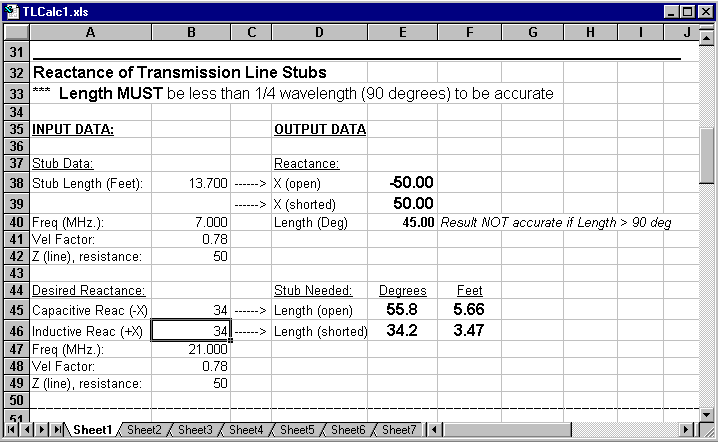Stub Matching

Using tuning stubs to match an antenna and transmission line is quite popular and useful. While the theory of stub matching is beyond the scope of this article, the following example illustrates how it may be performed using the AA3RL TLCalc spreadsheet.

We wish to match a 50 ohm feedline to an antenna with Z = 61 + j80. These values are entered as inputs. We immediately notice that the SWR is 3.88. Next, scan the third Column of the OUTPUT DATA to find where the parallel equivalent resistance, R(p), approaches 50 ohms. We see that this occurs somewhere between 80 and 90 degrees. A good initial guess would be 85 degrees. We also note that R(p) is not changing too quickly at this point so that measuring the stub insertion point will not be overly critical.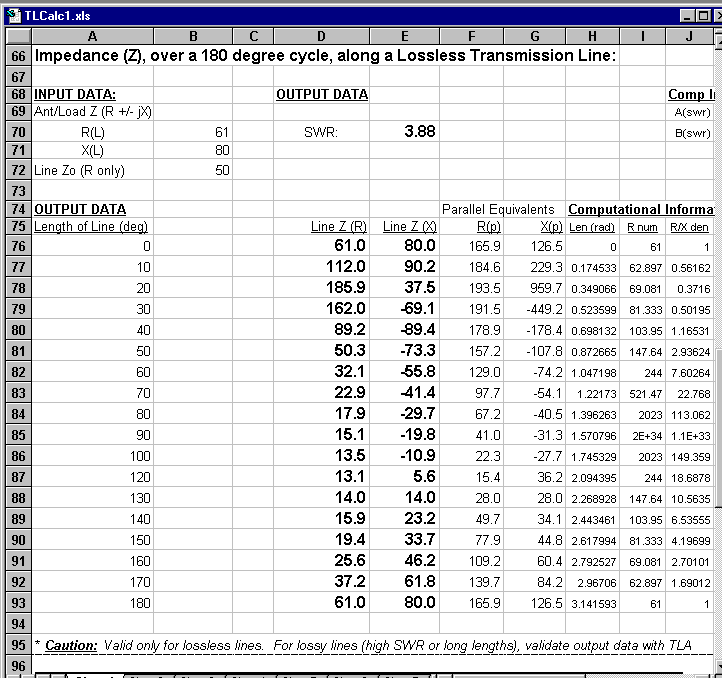Next move to the spreadsheet section for computing the impedance at a single point along a transmission line. Enter the same inputs: Z = 61 + j80, Frequency = 21 MHz., TL = 50 ohms, velocity factor 0.78. Start with the guess of 85 degree length. Adjust this value until you obtain a parallel equivalent R(p) = 50 ohms. This turns out to be 86 degrees, which at 21 MHz and vf 0.78 equals 8.73 feet. Thus the stub is inserted 8.73 feet from the antenna. The stub (or discrete component) must provide +34.44 ohms (inductive) reactance in order to cancel the - 34 ohms parallel equivalent (capacitive) reactance at the stub insertion point. This can be accomplished with a 3.74 foot shorted stub as shown above.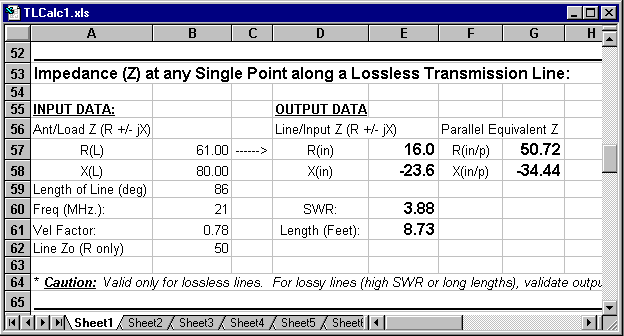Series Section Line Transformer (analytic solution) for impedance matching

While the theory of Series Section Line Transformer matching is beyond the scope of this article, the solution provided is analytic and straightforward. The same example used above for stub matching illustrates how it may be performed using a SSLT. In this case we elect to use 450 ohm ladder line with a vf = 0.95 for the matching section. The output indicates that a 0.96 foot length of ladder line should be inserted 5.03 feet from the antenna.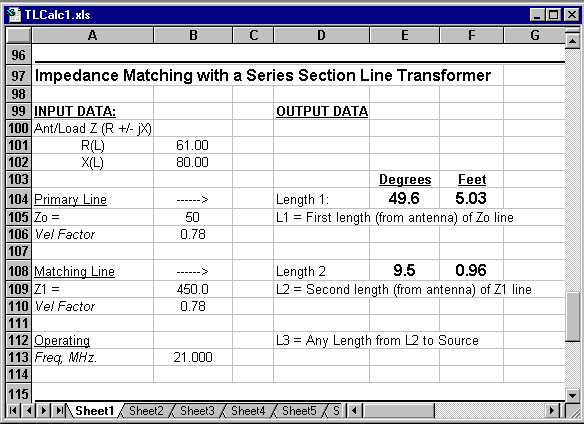Download:

The spreadsheet was created in Microsoft Excel 7.0 format, with the filename TLCalc1.xls. It has been zipped for storage and downloading. If it is updated in any significant way, the next version will be named "TLCalc2".

• Click here to download the AA3RL Transmission Line Calculator.
(TLCalc1.xls). Excel 7.0 spreadsheet format, (~10K zip file).This page is published by Mike Banz, AA3RL as a service to the Amateur Radio community.
Please distribute freely.

The author welcomes any questions, criticisms, or compliments via email.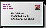email Mike with comments or questions.